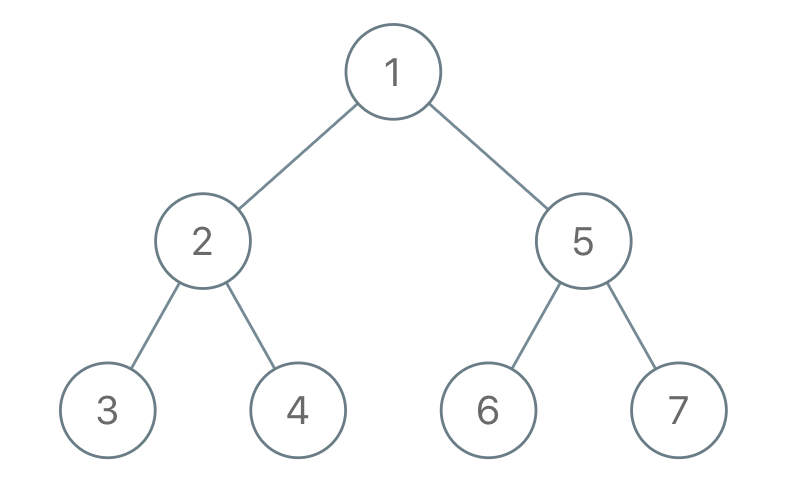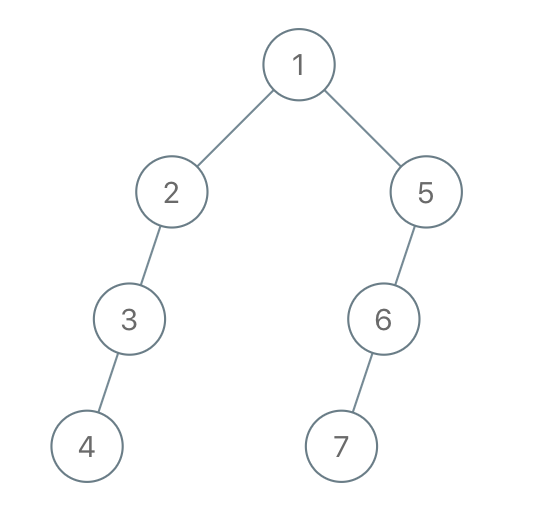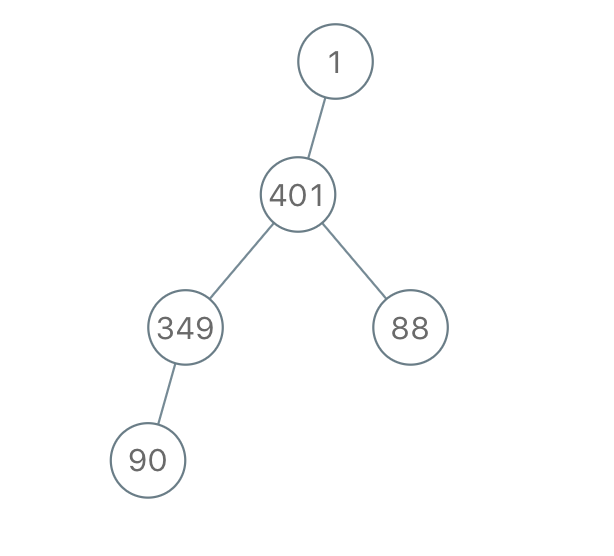# GeetCode Hub

We run a preorder depth-first search (DFS) on the `root` of a binary tree.

At each node in this traversal, we output `D` dashes (where `D` is the depth of this node), then we output the value of this node.  If the depth of a node is `D`, the depth of its immediate child is `D + 1`.  The depth of the `root` node is `0`.

If a node has only one child, that child is guaranteed to be the left child.

Given the output `traversal` of this traversal, recover the tree and return its `root`.

Example 1:```Input: traversal = "1-2--3--4-5--6--7"
Output: [1,2,5,3,4,6,7]
```

Example 2:```Input: traversal = "1-2--3---4-5--6---7"
Output: [1,2,5,3,null,6,null,4,null,7]
```

Example 3:```Input: traversal = "1-401--349---90--88"
Output: [1,401,null,349,88,90]
```

Constraints:

• The number of nodes in the original tree is in the range `[1, 1000]`.
• `1 <= Node.val <= 109`

/** * Definition for a binary tree node. * public class TreeNode { * int val; * TreeNode left; * TreeNode right; * TreeNode() {} * TreeNode(int val) { this.val = val; } * TreeNode(int val, TreeNode left, TreeNode right) { * this.val = val; * this.left = left; * this.right = right; * } * } */ class Solution { public TreeNode recoverFromPreorder(String traversal) { } }